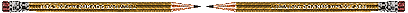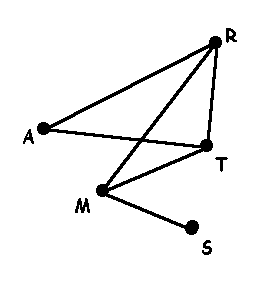Network TutorialWhat does Even and Odd Verticies mean ?

Even and Odd Verticies

Once you have the degree of the vertex you can decide if the vertex or node is even or odd.  If the degree of a vertex is even the vertex is called an even vertex.  On the other hand, if the degree of the vertex is odd, the vertex is called an odd vertex.Vertex Degree Even / Odd S 1 odd M 3 odd A 2 even R 3 odd T 3 odd

We can know look at if a graph is traversable by looking at the number of even and odd nodes.Graphs/networks       Degree of Vertex        Traversible       Euler Circuit       Eurler Path       Summary

 Main Page Glossary Activities Bridge Problem Applications Other Links